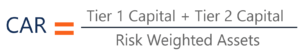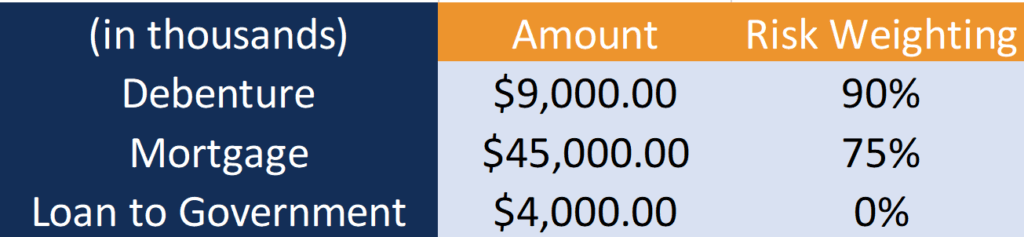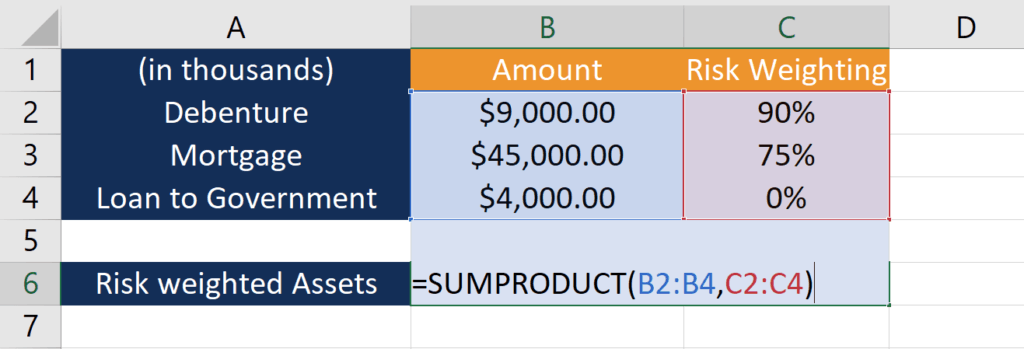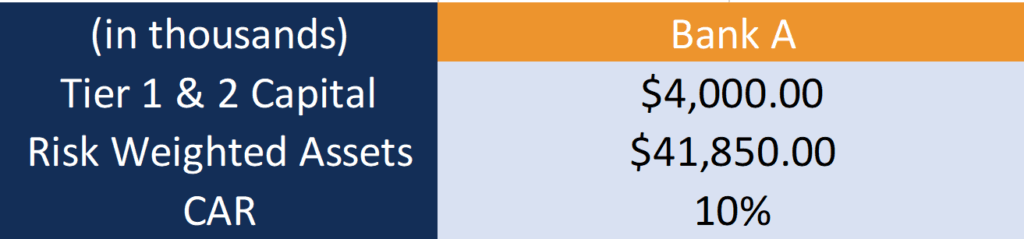Measures the ability of the bank to absorb losses

## What is the Capital Adequacy Ratio (CAR)?

The Capital Adequacy Ratio set standards for banks by looking at a bank’s ability to pay liabilities, respond to credit risks, and operational risks. A bank that has a good CAR has enough capital to absorb losses. Thus, it has less risk of becoming insolvent and losing depositor’s money. After the financial crisis in 2008, the Bank of International Settlements (BIS) began setting stricter CAR requirements to protect depositors.### Quick Summary Points

• Capital Adequacy Ratio (CAR) help makes sure banks have enough capital to protect depositors’ money.
• The formula for CAR is: (Tier 1 Capital + Tier 2 Capital) / Risk Weighted Assets
• Requirements set by the BIS changes and have become more strict in recent years.

### What is the Capital Adequacy Ratio Formula?

As shown below, the CAR Ratio formula is:CAR = (Tier 1 Capital + Tier 2 Capital) / Risk Weighted Assets

The Bank of International Settlements separates capital into Tier 1 and Tier 2 based on the function and quality of the capital. Tier 1 capital is the primary way to measure a bank’s financial health. It includes shareholder’s equity and retained earnings, which are disclosed on financial statements. As it is the core capital held in reserves, Tier 1 capital is capable of absorbing losses without impacting business operations. On the other hand, Tier 2 capital includes revalued reserves, undisclosed reserves, and hybrid security. Since this type of capital has lower quality, is less liquid, and is more difficult to measure, it is known as supplementary capital.

The bottom half of the equation is risk-weighted assets. Risk-weighted assets are the sum of a bank’s assets weighted by risk. Banks usually have different classes of assets such as cash, debentures, and bonds, and each class of asset is associated with a different weighting of risk. Risk weighting is decided based on the likelihood of an asset to decrease in value.

Asset classes that are safe, such as government debt, has a risk weighting close to 0%. Other assets backed by little or no collateral, such as debenture, has a higher risk weighting. This is because there is a higher likelihood the bank would not be able to collect the loan. Different risk-weighting can also be applied to the same asset class. For example, if a bank has lent money to three different companies, the loans can have different risk weighting based on the ability of the company to pay back the loan.

### Calculating the Capital Adequacy Ratio (CAR) – Worked Example

Let us look at an example of Bank A. Below is the information of the Bank A’s Tier 1 and 2 Capital and the risks associated with their assets.Bank A has three types of assets: Debenture, Mortgage, and Loan to the Government. To calculate the risk-weighted assets, the first step is to multiply the amount of each asset by the corresponding risk weighting:

• Debenture: \$9,000 * 90% = \$8,100
• Mortgage:  \$45,000 * 75% = \$33,750
• Loan to Government: \$4,000 * 0% = \$0

As the loan to the government carries no risk, it contributes \$0 to the risk-weighted assets.

The second step is to add the risk-weighted assets to arrive at the total:

• Risk-Weighted Assets: \$8,100 + \$33,750 + \$0 = \$41,850

The calculation can be easily done on Excel using the SUMPRODUCT function.The Capital Adequacy Ratio of Bank A is as follows :Where:

• CAR : \$4,000 / \$41,850 = 10%

As Bank A has a CAR of 10%, it has enough capital to cushion potential losses and protect depositor’s money.

### What are the Requirements?

Under the Basel III, all banks are required to have a Capital Adequacy Ratio of at least 8%. Since Tier 1 Capital is more important, banks are also required to have a minimum amount of this type of capital. Under Basel III, Tier 1 Capital divided by Risk Weighted Assets needs to be at least 6%.

• Bank Run
• Financial Statement for Banks
• Financial Intermediary